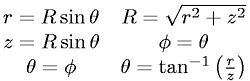Equations > Geometry > Coordinate Systems > Cylindrical to Spherical Coordinates (3-D)

Cylindrical to Spherical Coordinates (3-D)Latex Code:

MathML Code:

 $\begin{array}{ccc}\hfill r=R\mathrm{sin}\theta \hfill & \hfill R=\sqrt{{r}^{2}+{z}^{2}}\hfill & \hfill \hfill \\ \hfill z=R\mathrm{sin}\theta \hfill & \hfill \phi =\theta \hfill & \hfill \hfill \\ \hfill \theta =\phi \hfill & \hfill \theta ={\mathrm{tan}}^{-1}\left(\fracrz\right)\hfill & \hfill \hfill \end{array}$

MathType 5.0: